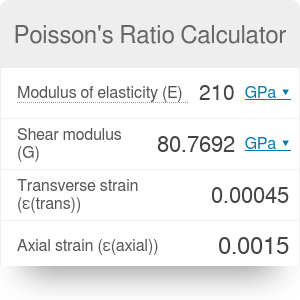Modulus of elasticity
GPa
Shear modulus
GPa
Transverse strain
Axial strain
Poisson's ratio

# Poisson's Ratio Calculator

By Bogna Haponiuk

This Poisson's ratio calculator is a tool that will help you determine the Poisson's ratio of any material. This calculator can work in two ways - either from the proportion of lateral and axial strain or from the relation between Young's modulus and shear modulus. Make sure to take a look at our torque calculator, too!

## Lateral strain and axial strain

Poisson's ratio is defined as the ratio between lateral strain and axial strain of a deformed object. Imagine it like this: if you compress a piece of rubber from above, it will "flow" sideways, increasing its width. On the other hand, if you do the same with cork, you will discover that it simply changed its volume and almost no increase in width was observed. Rubber is an example of a material with high Poisson's ratio, while cork - with low Poisson's ratio.

The Poisson's ratio calculator uses the following formula:

`ν = - ε(trans) / ε(axial)`

where

• `ν` is the Poisson's ratio (dimensionless);
• `ε(trans)` is the transverse (lateral) strain - the relative change in dimension perpendicular to the direction of force;
• `ε(trans)` is the axial strain - the relative change in dimension parallel to the direction of force.

We always assume tension (stretching) to be positive and compression to be negative. Notice that Poisson's ratio will always be positive in result - it is impossible to have a material that, when compressed in one direction, will automatically compress in the transverse direction as well. Most materials have Poisson's ratio between 0 and 0.5, where 0.5 corresponds to a perfectly incompressible material (one that doesn't change its volume).

## Young's modulus and shear modulus

You can also use our Poisson's ratio calculator to find Poisson's ratio basing on the values of shear modulus and modulus of elasticity. These three parameters are related with the following equation:

`E = 2 * G * (1 + ν)`

where

• `ν` is the Poisson's ratio;
• `E` is the modulus of elasticity (Young's modulus) in GPa;
• `G` is the shear modulus in GPa.

This equation is true only for isotropic materials.

If you want to find out more about the Young's modulus, check out our stress calculator.

Bogna Haponiuk

## Get the widget!

Poisson's Ratio Calculator can be embedded on your website to enrich the content you wrote and make it easier for your visitors to understand your message.

It is free, awesome and will keep people coming back!